# How To Find The Time Constant in RC and RL Circuits

Contents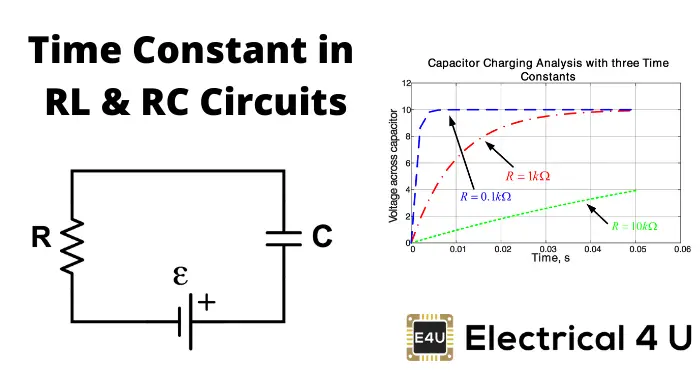## What is the Time Constant?

The time constant – usually denoted by the Greek letter τ (tau) – is used in physics and engineering to characterize the response to a step input of a first-order, linear time-invariant (LTI) control system. The time constant is the main characteristic unit of a first-order LTI system.

The time constant is commonly used to characterize the response of an RLC circuit.

To do this, let us derive the time constant for an RC circuit, and the time constant for an RL circuit.

## Time Constant of an RC Circuit

Let us take a simple RC circuit, as shown below.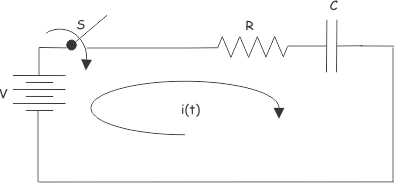Let us assume the capacitor is initially uncharged and the switch S is closed at time t = 0. After closing the switch, electric current i(t) starts flowing through the circuit. Applying Kirchhoff Voltage Law in that single mesh circuit, we get,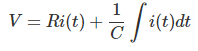Differentiating both sides with respect to time t, we get,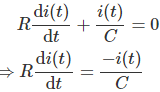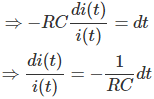Integrating both sides we get,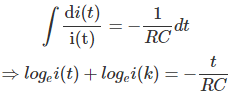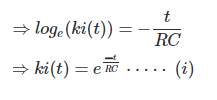Now, at t = 0, the capacitor behaves as a short circuit, so, just after closing the switch, the current through the circuit will be,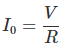Now, putting this value in equation (I), we get,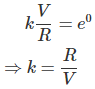Putting the value of k at equation (I), we get,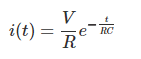Now, if we put t = RC in the final expression of circuit current i(t), we get,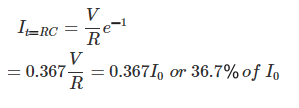From the above mathematical expression, it is clear that RC is the time in second during which the current in a charging capacitor diminishes to 36.7 percent from its initial value. Initial value means current at the time of switching on the unchanged capacitor.

This term is quite significant in analyzing the behavior of capacitive as well as inductive circuits. This term is known as the time constant.

So time constant is the duration in seconds during which the current through a capacities circuit becomes 36.7 percent of its initial value. This is numerically equal to the product of resistance and capacitance value of the circuit. The time constant is normally denoted by τ (tau). So,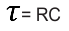In a complex RC circuit, the time constant will be the equivalent resistance and capacitance of the circuit.

Let us discuss the significance of the time constant in more detail. To do this, let us first plot current i(t).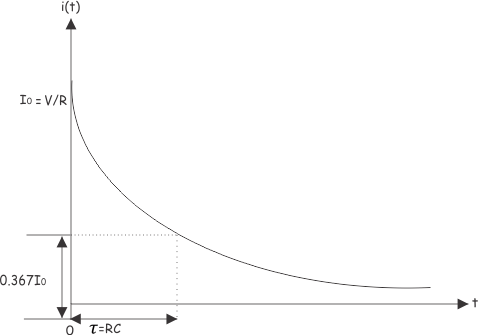At t = 0, the current through the capacitor circuit is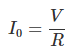At t = RC, the current through the capacitor is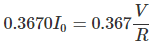Let us consider another RC circuit.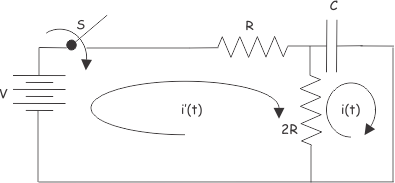Circuit equations using KVL of the above circuits are,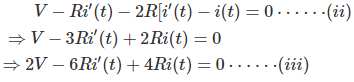and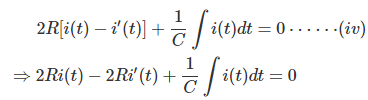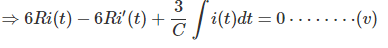From (iii) and (v)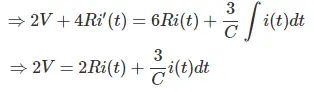Differentiating both sides with respect to time t, we get,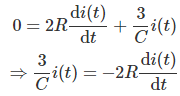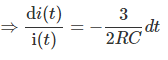Integrating both sides we get,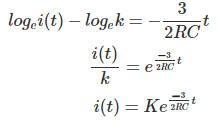At t = 0,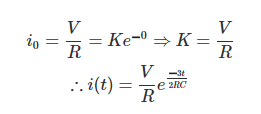The time constant of this circuit would be 2RC/3 sec. Now, the equivalent resistance of the circuit is,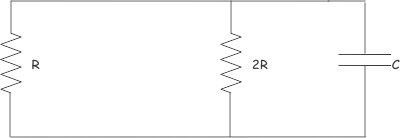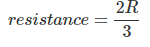The time constant of the circuit has become.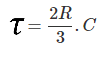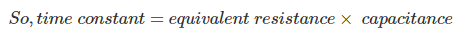## Time Constant of an RL Circuit

Let us consider an example of a series RL circuit.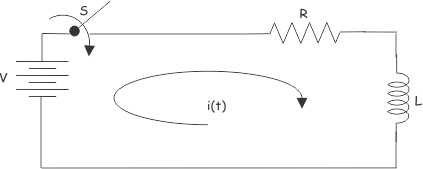Applying Kirchhoff Voltage Law in the above circuit. We get,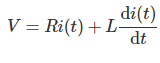The equation can also be solved Laplace Transformation technique. For that, we have to take Laplace Transformation of the equation at both sides,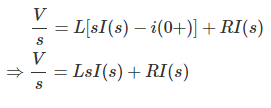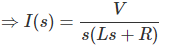Hence, in this equation.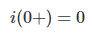Since the current just after the switch is on, the current through the inductor will be zero.
Now,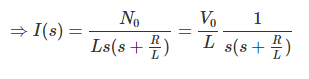Taking inverse Laplace of the above equation, we get,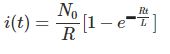Now, if we put,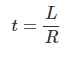We get,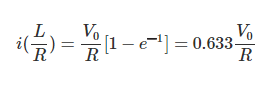At the RL circuit, at time = L/R sec, the current becomes 63.3% of its final steady-state value. The L/R is known as the time constant of an LR circuit. Let us plot the current of the inductor circuit.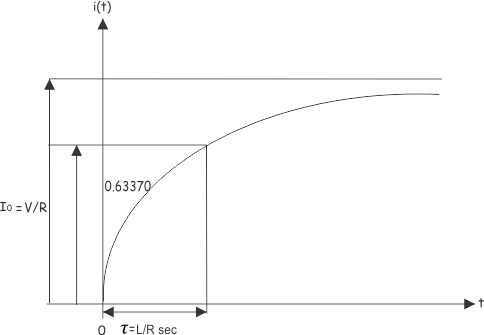The time constant of an LR circuit is the ratio of inductance to the resistance of the circuit. Let us take another.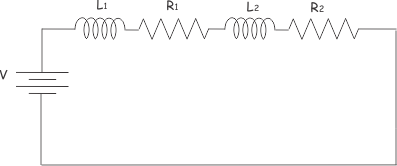This circuit can be redrawn as,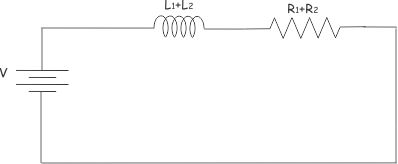So, the time constant of the circuit would be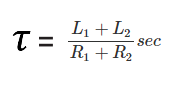Want To Learn Faster? 🎓
Get electrical articles delivered to your inbox every week.
No credit card required—it’s 100% free.